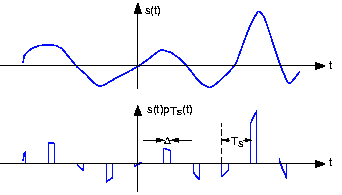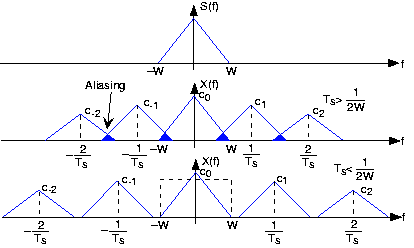# The sampling theorem

 Page 1 / 1
Converting between a signal and numbers.

## Analog-to-digital conversion

Because of the way computers are organized, signal must be represented by a finite number of bytes. This restrictionmeans that both the time axis and the amplitude axis must be quantized : They must each be a multiple of the integers. We assume that we do not use floating-point A/D converters. Quite surprisingly, the Sampling Theorem allows us to quantizethe time axis without error for some signals. The signals that can be sampled without introducingerror are interesting, and as described in the next section, we can make a signal "samplable" by filtering. In contrast,no one has found a way of performing the amplitude quantization step without introducing an unrecoverable error.Thus, a signal's value can no longer be any real number. Signals processed by digital computers must be discrete-valued : their values must be proportional to the integers. Consequently, analog-to-digital conversion introduces error .

## The sampling theorem

Digital transmission of information and digital signal processing all require signals to first be "acquired" by acomputer. One of the most amazing and useful results in electrical engineering is that signals can be converted from afunction of time into a sequence of numbers without error : We can convert the numbers back into the signal with (theoretically) no error. Harold Nyquist, a Bell Laboratories engineer, first derived this result, known as the Sampling Theorem, in the1920s. It found no real application back then. Claude Shannon , also at Bell Laboratories, revived the result once computerswere made public after World War II.

The sampled version of the analog signal $s(t)$ is $s(n{T}_{s})$ , with ${T}_{s}$ known as the sampling interval . Clearly, the value of the original signal at the sampling times ispreserved; the issue is how the signal values between the samples can be reconstructed since they are lost in the samplingprocess. To characterize sampling, we approximate it as the product $x(t)=s(t){P}_{{T}_{s}}(t)$ , with ${P}_{{T}_{s}}(t)$ being the periodic pulse signal. The resulting signal, as shown in [link] , has nonzero values only during the time intervals $\left(n{T}_{s}-\frac{\Delta }{2} , n{T}_{s}+\frac{\Delta }{2}\right)$ , $n\in \{\dots , -1, 0, 1, \dots \}$ .The waveform of an example signal is shown in the top plot and its sampled version in the bottom. For our purposes here, we center the periodic pulse signal about the origin so that its Fourier series coefficients arereal (the signal is even).
${p}_{{T}_{s}}(t)=\sum_{k=()}$ c k 2 k t T s
${c}_{k}=\frac{\sin \left(\frac{\pi k\Delta }{{T}_{s}}\right)}{\pi k}$
If the properties of $s(t)$ and the periodic pulse signal are chosen properly, we can recover $s(t)$ from $x(t)$ by filtering.

To understand how signal values between the samples can be "filled" in, we need to calculate the sampled signal'sspectrum. Using the Fourier series representation of the periodic sampling signal,

$x(t)=\sum_{k=()}$ c k 2 k t T s s t
Considering each term in the sum separately, we need to know the spectrum of the product of the complex exponential and thesignal. Evaluating this transform directly is quite easy.
$\int_{()} \,d t$ s t 2 k t T s 2 f t t s t 2 f k T s t S f k T s
Thus, the spectrum of the sampled signal consists of weighted (by the coefficients ${c}_{k}$ ) and delayed versions of the signal's spectrum( [link] ).
$X(f)=\sum_{k=()}$ c k S f k T s
In general, the terms in this sum overlap each other in thefrequency domain, rendering recovery of the original signal impossible. This unpleasant phenomenon is known as aliasing .The spectrum of some bandlimited (to W Hz) signal is shown in the top plot. If the sampling interval T s is chosen too large relative to the bandwidth W , aliasing will occur. In the bottom plot, the samplinginterval is chosen sufficiently small to avoid aliasing. Note that if the signal were not bandlimited, thecomponent spectra would always overlap. If, however, we satisfy two conditions:
• The signal $s(t)$ is bandlimited —has power in a restricted frequency range—to $W$ Hz, and
• the sampling interval ${T}_{s}$ is small enough so that the individual components in thesum do not overlap— ${T}_{s}< 1/2W$ ,
aliasing will not occur. In this delightful case, we can recover the original signal by lowpass filtering $x(t)$ with a filter having a cutoff frequency equal to $W$ Hz. These two conditions ensure the ability to recover abandlimited signal from its sampled version: We thus have the Sampling Theorem .

The Sampling Theorem (as stated) does not mention the pulse width $\Delta$ . What is the effect of this parameter on our ability torecover a signal from its samples (assuming the Sampling Theorem's two conditions are met)?

The only effect of pulse duration is to unequally weight the spectral repetitions. Because we are only concernedwith the repetition centered about the origin, the pulse duration has no significant effect on recovering a signalfrom its samples.

The frequency $\frac{1}{2{T}_{s}}$ , known today as the Nyquist frequency and the Shannon sampling frequency , corresponds to the highest frequency at which a signal can contain energy andremain compatible with the Sampling Theorem. High-quality sampling systems ensure that no aliasing occurs byunceremoniously lowpass filtering the signal (cutoff frequency being slightly lower than the Nyquist frequency) beforesampling. Such systems therefore vary the anti-aliasing filter's cutoff frequency as the sampling rate varies. Because such quality featurescost money, many sound cards do not have anti-aliasing filters or, for that matter, post-samplingfilters. They sample at high frequencies, 44.1 kHz for example, and hope the signal contains no frequencies above theNyquist frequency (22.05 kHz in our example). If, however, the signal contains frequencies beyond the sound card's Nyquistfrequency, the resulting aliasing can be impossible to remove.

To gain a better appreciation of aliasing, sketch the spectrum of a sampled square wave. For simplicityconsider only the spectral repetitions centered at $-\left(\frac{1}{{T}_{s}}\right)$ , $0$ , $\frac{1}{{T}_{s}}$ . Let the sampling interval ${T}_{s}$ be 1; consider two values for the square wave's period:3.5 and 4. Note in particular where the spectral lines go as the period decreases; some will move to the left andsome to the right. What property characterizes the ones going the same direction?

The square wave's spectrum is shown by the bolder set of lines centered about the origin. The dashed linescorrespond to the frequencies about which the spectral repetitions (due to sampling with ${T}_{s}=1$ ) occur. As the square wave's period decreases, the negativefrequency lines move to the left and the positive frequency ones to the right.

If we satisfy the Sampling Theorem's conditions, the signalwill change only slightly during each pulse. As we narrow the pulse, making $\Delta$ smaller and smaller, the nonzero values of the signal $s(t){p}_{{T}_{s}}(t)$ will simply be $s(n{T}_{s})$ , the signal's samples . If indeed the Nyquist frequency equals the signal's highest frequency, at least twosamples will occur within the period of the signal's highest frequency sinusoid. In these ways, the sampling signalcaptures the sampled signal's temporal variations in a way that leaves all the original signal's structure intact.

What is the simplest bandlimited signal? Using this signal, convince yourself that less than twosamples/period will not suffice to specify it. If the sampling rate $\frac{1}{{T}_{s}}$ is not high enough, what signal would your resulting undersampled signal become?

The simplest bandlimited signal is the sine wave. At theNyquist frequency, exactly two samples/period would occur. Reducing the sampling rate would result in fewersamples/period, and these samples would appear to have arisen from a lower frequency sinusoid.

Application of nanotechnology in medicine
what is variations in raman spectra for nanomaterials
I only see partial conversation and what's the question here!
what about nanotechnology for water purification
please someone correct me if I'm wrong but I think one can use nanoparticles, specially silver nanoparticles for water treatment.
Damian
yes that's correct
Professor
I think
Professor
what is the stm
is there industrial application of fullrenes. What is the method to prepare fullrene on large scale.?
Rafiq
industrial application...? mmm I think on the medical side as drug carrier, but you should go deeper on your research, I may be wrong
Damian
How we are making nano material?
what is a peer
What is meant by 'nano scale'?
What is STMs full form?
LITNING
scanning tunneling microscope
Sahil
how nano science is used for hydrophobicity
Santosh
Do u think that Graphene and Fullrene fiber can be used to make Air Plane body structure the lightest and strongest. Rafiq
Rafiq
what is differents between GO and RGO?
Mahi
what is simplest way to understand the applications of nano robots used to detect the cancer affected cell of human body.? How this robot is carried to required site of body cell.? what will be the carrier material and how can be detected that correct delivery of drug is done Rafiq
Rafiq
if virus is killing to make ARTIFICIAL DNA OF GRAPHENE FOR KILLED THE VIRUS .THIS IS OUR ASSUMPTION
Anam
analytical skills graphene is prepared to kill any type viruses .
Anam
what is Nano technology ?
write examples of Nano molecule?
Bob
The nanotechnology is as new science, to scale nanometric
brayan
nanotechnology is the study, desing, synthesis, manipulation and application of materials and functional systems through control of matter at nanoscale
Damian
Is there any normative that regulates the use of silver nanoparticles?
what king of growth are you checking .?
Renato
What fields keep nano created devices from performing or assimulating ? Magnetic fields ? Are do they assimilate ?
why we need to study biomolecules, molecular biology in nanotechnology?
?
Kyle
yes I'm doing my masters in nanotechnology, we are being studying all these domains as well..
why?
what school?
Kyle
biomolecules are e building blocks of every organics and inorganic materials.
Joe
anyone know any internet site where one can find nanotechnology papers?
research.net
kanaga
sciencedirect big data base
Ernesto
Introduction about quantum dots in nanotechnology
hi
Loga
what does nano mean?
nano basically means 10^(-9). nanometer is a unit to measure length.
Bharti
Difference between extinct and extici spicies
Got questions? Join the online conversation and get instant answers!By OpenStaxBy Richley CrapoByBy Brooke DelaneyByBy Brooke DelaneyBy OpenStaxBy Stephen VoronBy Cath YuBy Stephen Voron